# Electronic Configuration

## Electronic configuration of Elements

• Electronic configuration or electron configuration is the Arrangement of electrons in different orbitals of an atom.
• The basic rules which govern the filling of different atomic orbitals are Aufbau Principle which states that atomic orbital are filled in increasing order of enery, Pauli Exclusion Principle which states that an orbital can have maximum 2 electron only and Hund's rule of maximum multiplicity which states that pairing or doubling of electrons in the orbitals belonging to the same subshell (p, d or f) does not take place until each orbital is single occupied
• The electronic configuration of different atoms can be represented in two ways.
a. $s^a ,p^b d^c$ ...... notation.

In this representation,the subshell is represented by the respective letter symbol and the number of electrons present in the subshell is depicted, as the super script, like a, b, c, ... etc. The similar subshell represented for different shells is differentiated by writing the principal quantum number before the respective subshell.
For example, Electronic configuration of Na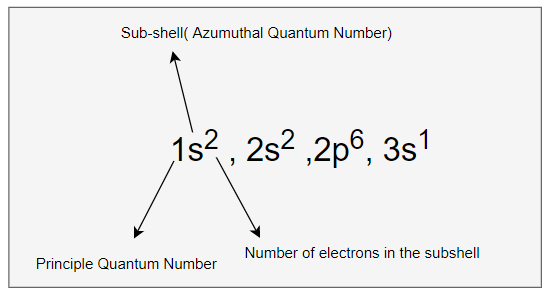b. Orbital diagram: each orbital of the subshell is represented by a box and the electron is represented by an arrow (↑) a positive spin or an arrow (↓) a negative spin. The advantage of second notation over the first is that it represents all the four quantum numbers.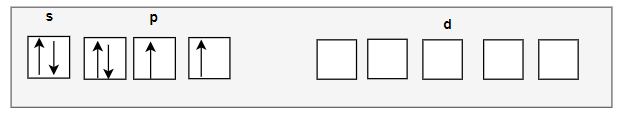For example, Electronic configuration of Na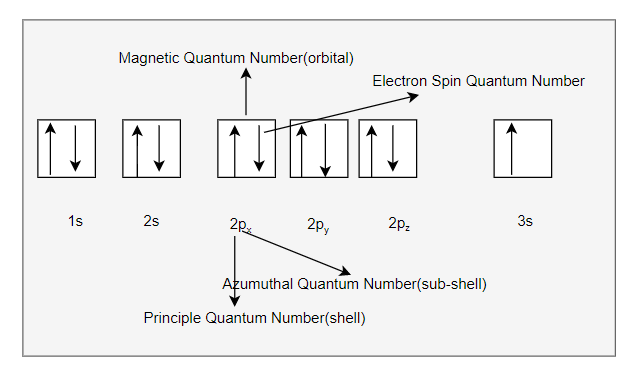Few important note
(1) the electron in the  completely filled shell are called core electrons
(2) The electrons that are added to the electronic shell with the highest principle quantum number is called Valence electrons

## Why Electronic configuration of atoms is required

The knowledge of Electronic configuration of atoms is required to help us understand the chemical properties of the various elements

## How to obtain the electronic configuration

1. First obtain the Atomic Number of the element. The atomic number in neutral atom represent the Number of electrons
2. Start filling the electron in the subshell in below order given by Aufbau
1s, 2s, 2p, 3s, 3p, 4s, 3d, 4p, 5s, 4d, 5p, 6s,4f,5d, 6p, 7s...
3. We will be putting max 2 electron per orbital in the subshell. So s-subshell can have max 2 electron, p-subshell have 6 electrons, d-subshell have 10 electrons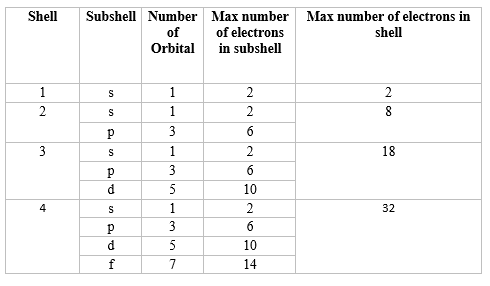4. if we are writing down the orbital diagram, we will need to remember Pauli Exclusion Principle and Hunds rule and show electrons in orbital with correct spin and correct pairing
5. We will try to go with both notation and orbital diagram to give the correct picture
6. There are exception to the rules above. Such as seen in few atoms like chromium and copper. chromium and copper have five and ten electrons in 3d orbitals rather than four and nine as their position would have indicated with two-electrons in the 4s orbital. The reason is that fully filled orbitals and half-filled orbitals have extra stability (that is, lower energy). Thus p3, p6, d5, d10,f7, f14 etc. configurations, which are either half-filled or fully filled, are more stable. Chromium and copper therefore adopt the d5 and d10 configuration
7.Sometime the total configuration can be fairly long(incase of large atomic number).In such cases, you can use the abbreviated notation. You can use the previous noble gas to abbreviate the configuration in such cases You just have to finish the configuration from where the noble gas leaves it
Example
Na -> [Ne] $3s^1$
Ar -> [Ne] $3s^2,3p^6$

## Example of electron configuration

1. H(Z=1)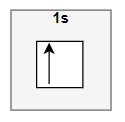2. He(Z=2)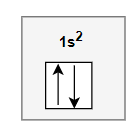3. Eight elements
Beryllium (Be,$1s^2 ,2s^2$ ) , Lithium (Li,$1s^2,2s^1$, boron (B, $1s^2,2s^2,2p^1$), carbon (C, $1s^2,2s^2,2p^2$), nitrogen (N, $1s^2,2s^2,2p^3$), oxygen (O, $1s^2,2s^2,2p^4$), fluorine (F, $1s^2,2s^2,2p^5$) and neon (Ne, $1s^2,2s^2,2p^6$)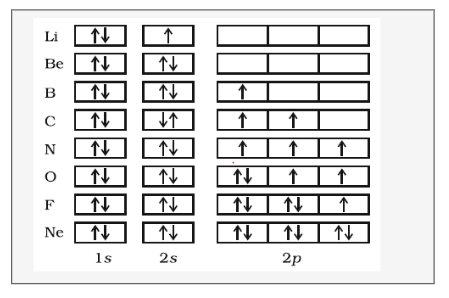We can write the electronic connfigurtion of other elements in the same manner

## Example of exceptional electron configuration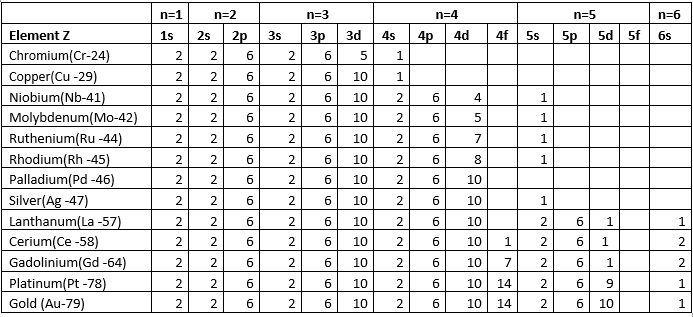## Stability of completely filled and half filled subshells:

Some of the exception in electronic configuration can be explained with below concept
Symmetrical distribution of electrons: the completely filled or half-filled sub-shells have  symmetrical distribution of electrons in them and are more stable.
Exchange energy-The two or more electrons with the same spin present in the degenerate orbitals of a sub-shell can exchange their position and the energy released due to this exchange is called exchange energy. The number of exchanges is maximum when the subshell is either half filled or completely filled. As a result the exchange energy is maximum and so is the stability.

## Electronic configuration of Cation & Anions

• The electronic configuration of the ions may be written almost similar to these of the atpms in case of negatively charged ions ,the extra electrons equal to the negative charge is added to the appropiate orbitals.
• The electrons equal to the charge on the iob are removed from the neutral atom in case of positively charged ions. But it must be remembered that electrons with the highest value of principle quantum number are removed first.If two or more subshell are there for such n value,then the subshell with higher value of azumutal quantum number(l) loses the electron first

Question 1
Write the electronic Configuration for the following atomic numbers
(a) 23
(b) 30
(c) 19
(d) 38
(e) 25
Solution
We know the rules of filling the electron in the orbit are
(a) As per Aufbau rule or Principle,the orbitals are filled in increasing order of their energies.
1s, 2s ,2p, 3s ,3p ,4s ,3d ,4p, 5s,4d,5p,4f,5d,6p
(b) There can be maximum two electrons in the orbital
(c) pairing of electrons in the orbital’s belonging to same sub – shell [ p, d, f], does not take place until each orbital gets singly occupied
So p subshell can be have maximum 6 electrons and d-subshell have 10 electrons
So electronic configuration can be written as
(a) 1s2 , 2s2, 2p6 ,3s2 ,3p6,4s2 ,3d3
(b) 1s2 , 2s2, 2p6 ,3s2 ,3p6,4s2 ,3d10
(c) 1s2 , 2s2, 2p6 ,3s2 ,3p6,4s1
(d) 1s2 , 2s2, 2p6 ,3s2 ,3p6,4s2 ,3d10 ,5s2 ,4p6
(e) 1s2 , 2s2, 2p6 ,3s2 ,3p6,4s2 ,3d5
Question 2
Give the no. of unpaired electrons in Iron (Fe26)
Solution
Electronic Configuration of Fe26 = 1s2 ,2s2,2p6 ,3s2, 3p6 ,4s2,3d6
So, there will be ‘4’ unpaired electrons.
Question 3
Give the no. of unpaired e- in Fe +3
Solution
Electronic Configuration of Fe26 = 1s2 ,2s2,2p6 ,3s2, 3p6 ,4s2,3d6
Now For Fe+3
E. C. is: 1s2 2s2 2p6 3p2 3p6 3d5
Question 4
(i) Write E. C. of 19th e- in chromium (24)
(ii) Give all 4 values of quantum no. for 19th e-.
Solution
Predicted E. C. of chromium: -
=1s2 2s2 2p6 3s2 3p6 4s2 3d4
E. C. of chromium: - = 1s2 2s2 2p6 3s2 3p6 4s1 3d5
The reason is half filled subshell are more stable So, configuration of 19th electrons:
4s1   n=4,l=0,ml =0, ms =1/2

Question 4
How many unpaired electrons are present in cobalt [Co] metal
(a) 2
(b) 3
(c) 4
(d) 7
Solution
3 unpaired electron are present in cobalt metal.

Question 5
The number of unpaired electrons in nitrogen is
(a) 1
(b) 3
(c) 2
(d) None of these
Solution
3 unpaired electron are present in nitrogen.